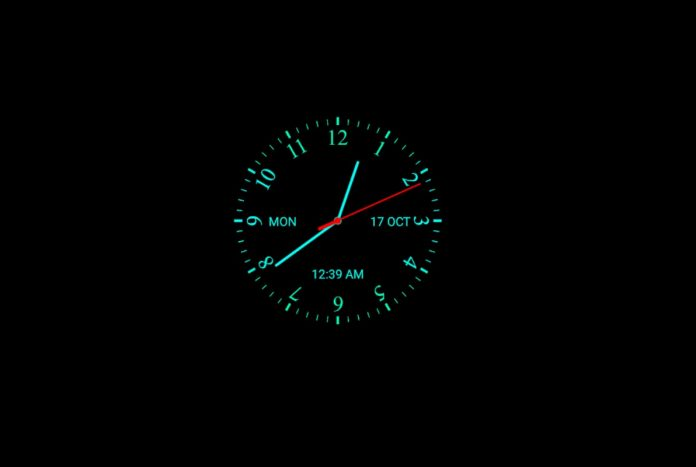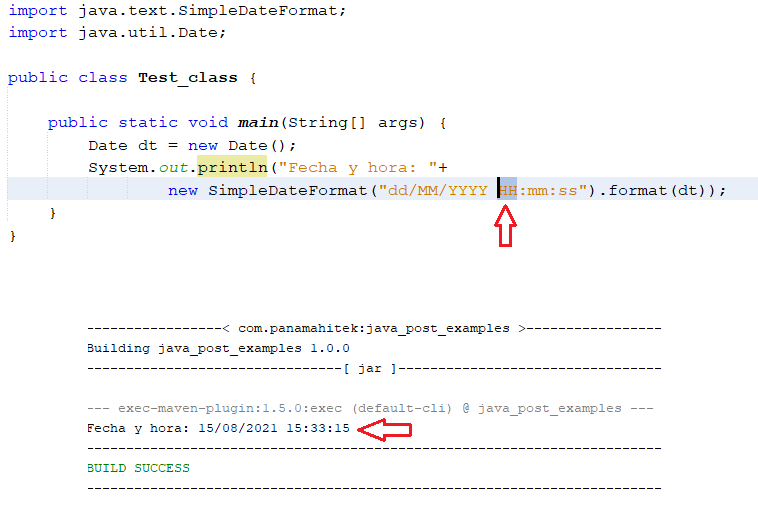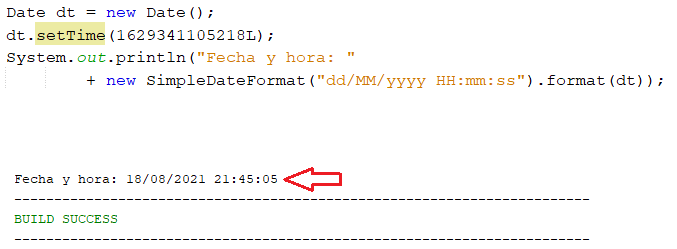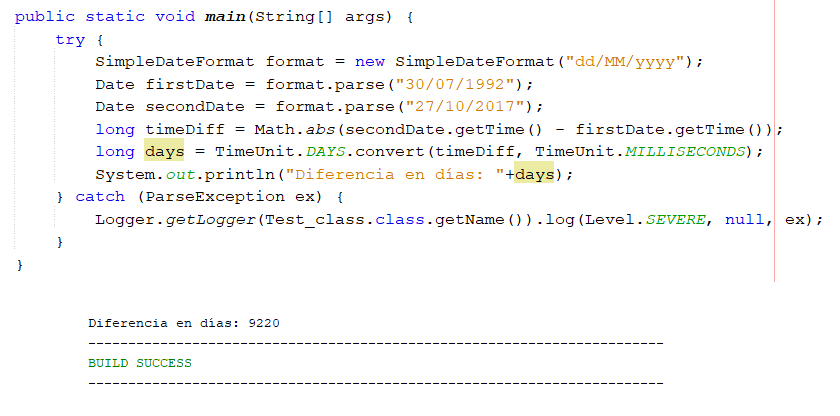# Time and Dates in Java

0
54In programming, the management of time and dates in Java is a very interesting topic, since each programmer will have their own way of doing it. I will write this post based on my experience in the development of programming algorithms.

In Java there are several types of “primitive data”, among which is the Date class. To create an object of class Date, we do the following:

In this example, the dt object is an instance of the Date class. What information does that object contain? Well, one way to extract that information is through the SimpleDateFormat class.

In the following example I am going to create an object of the Date class and I am going to print the content of that object to the console. The code is the following:

The result of running this script is:

Let me explain what happened:

• When you create an object of class Date (such as dt), that object automatically assumes the value of the instant of time in which the code is executed. In my case, I ran this code on August 15, 2021 at 10 a.m., 24 minutes and 8 seconds. If I run the code again, a different result will appear in the console.
• To extract the data from the dt object, the SimpleDateFormat class is used, which has the format() method. This method converts the Date class objects into character strings (String) following the format provided in the class constructor. In this case the parameter I used in the class constructor was “dd/MM/yyyy hh:mm:ss“. Those parameters mean: “day/month/year hour:minutes:seconds”.

Here I have the parameters that can be used in the constructor of the SimpleDateFormat class:

Param. Description Data type Example
`G` Era designator Text `AD`
`y` Year Year `1996``96`
`Y` Year Year `2009``09`
`M` Month of the year Month `July``Jul``07`
`w` Week of the year Number `27`
`W` Week of the month Number `2`
`D` Day of the year Number `189`
`d` Day of the month Number `10`
`F` Day of the week in month Number `2`
`E` Name of the day Text `Tuesday``Tue`
`u` Number of day in week Number `1`
`a` AM/PM mark Text `PM`
`H` Hour (0-23) Number `0`
`k` Hour (1-24) Number `24`
`K` AM/PM Hour (0-11) Number `0`
`h` AM/PM Hour (1-12) Number `12`
`m` Minutes in hour Number `30`
`s` Seconds in a minute Number `55`
`S` Miliseconds Number `978`
`z` Time Zone General time zone `Pacific Standard Time``PST``GMT-08:00`
`Z` Time Zone RFC 822 time zone `-0800`
`X` Time Zone ISO 8601 time zone `-08``-0800``-08:00`

With these parameters we can make the date and time in Java adopt the format we need. For example, if in the example shown above I want the time to be in 24 hour format (0 being 12 AM and 23 11 PM), the code to use is the following:

I just changed the “hh” to “HH” in the constructor parameters of the SimpleDateFormat class. The result is:With the parameters shown in the table above we can give the form we want to the dates in Java. The “:” and “/” are not part of the parameters, but instead format the text output in a format that is easy for users to understand.

##### Create objects with specific dates

If we want to create an object with a specific date we will have to use the parse() method of the SimpleDateFormat class. With that method you can convert Strings to Date, which is very useful in some cases.

For example, if we want to create an object of the Date class with the date October 27, 2017 at 12:03 AM, we will do the following:

This statement could throw an error, so it must be inside a Try Catch. With the code shown here we will have an object of the Date class that contains the date that we specify in text format. It is important that the date we provide has the same format as the parameters we use in the constructor of the SimpleDateFormat class.

##### Timestamps in Java

As we have already seen, the use of the Date class is not that simple. Both days and hours can be stored in a date object. We know as a date a combination of day / month / year. Hours are a combination of hours:minutes:seconds. If necessary, we could create a Date object with the complete information of an “instant” of time. This would be the combination of day, month, year, hour, minutes, seconds, and milliseconds.

A lot of information can be stored in a Date object, but it can be complicated. Sometimes it is easier to convert dates to a whole number and to work with numbers rather than all components of the dates. For this there are timestamps. The so-called “epochs”.

An epoch is a number that represents an instant of time. It is made up of the number of milliseconds that have elapsed since 00:00:00 on January 1, 1970. Of course, epochs are very large numbers.

For example, the epoch of the moment I am writing this paragraph is 1629341105218, given in milliseconds. I am going to try to convert that number to a date in Java. The code is the following:

This code should produce the following output:The “L” that is placed at the end of the number of milliseconds is to indicate that it is a Long type data.

With dates in epoch format, it is easier to do operations with dates, such as adding or subtracting days or hours, minutes or seconds. For example, if I want to subtract one day from the date in the previous example, I would do the following:

By subtracting 864000000 from the epoch, I am taking away the number of milliseconds that a day contains. We can also subtract or add hours (subtracting 3600000), minutes (60000), seconds (1000), weeks, months, etc.

Timespamps in epoch format are also very useful in database management, since it is easier to save a number than a set of characters that need to be interpreted in a specific way. The epoch is one of the most universal time notations that can be used in software development and information management.

Difference between dates

To calculate the difference between dates in Java there is a great variety of techniques, both with native resources and with external libraries. Using the Date and SimpleDateFormat classes we can achieve this easily. Let’s see an example:

The result of this code is the following:Time differences can be expressed in days (see example), hours, minutes, seconds, milliseconds, and nanoseconds. For more possibilities in calculating the difference between dates, external libraries designed for this purpose can be used.

I hope the information presented here is useful to you. Any questions or suggestions can be sent to me through the comment box.